100 Best Fuzzy Logic Videos

Fuzzy Logic

Fuzzy Logic & Dialog Systems 2014

fuzzylogicMerge1 [54x Apr 2015]

• tutorial on fuzzylite: a fuzzy logic control library in C++

tutorial on fuzzylite: a fuzzy logic control library in C++ From: Shankar Puja Views: 301 0 ratings Time: 27:34 More in People & Blogs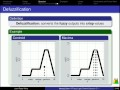• Synopsis | An Introduction To Fuzzy Logic For Practical Applications

Synopsis | An Introduction To Fuzzy Logic For Practical Applications THE SYNOPSIS OF YOUR FAVORITE BOOK =— Where to buy this book? ISBN: 9780387948072 Book Synopsis of An Introduction to Fuzzy Logic for Practical Applications by Kazuao Tanaka … From: Maminha Books Views: 0 0 ratings Time:…• Automatic Cleaning Robot Based on Fuzzy Logic

Automatic Cleaning Robot Based on Fuzzy Logic using PIC18F458, Accelerometer (ADXL335), IR Sensor,Dust sensor, Stepper Motor and DC motor with Encoder. From: MRITYUNJAY KUMAR Views: 14 1 ratings Time: 03:02 More in People & Blogs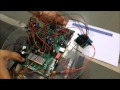• Wall following robot using fuzzy logic

Wall following robot using fuzzy logic Wall following robot using fuzzy logic. From: Pengxiang Jin Views: 3 0 ratings Time: 04:47 More in Science & Technology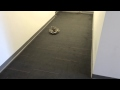• Lecture 6 : MATLAB Fuzzy Logic Toolbox

Lecture 6 : MATLAB Fuzzy Logic Toolbox MATLAB Fuzzy Logic Toolbox Introduction Graphical User Interface (GUI) Tools Example: Dinner for two. From: Lalit Patil Views: 15 0 ratings Time: 01:41 More in Music• Fuzzy Logic FMS Lego Robotics Competition 2015

Fuzzy Logic FMS Lego Robotics Competition 2015 Programmed by an EV3, Option 1 Fuzzy Logic from FMS wins 1st place in the 2015 robotics competition. From: 1komotion Views: 7 0 ratings Time: 00:18 More in People & Blogs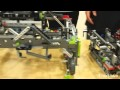• Introduction to Fuzzy Logic

Introduction to Fuzzy Logic This video is made to fulfill the requirement of Assignment #1 for course AEEM 6096 at University of Cincinnati. This will serve as an introduction to Fuzzy Logic and control systems. From: Anuja Papriwal Views: 17 1 ratings Time: 04:30 More in People &…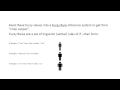• Introduction to Fuzzy Logic !

Introduction to Fuzzy Logic ! This video talks about Fuzzy Logic, its uses and future applications. From: sudhir rssk Views: 11 1 ratings Time: 02:55 More in People & Blogs• An Introduction to Fuzzy Logic

An Introduction to Fuzzy Logic This video quickly describes Fuzzy Logic and its uses for assignment 1 of Dr. Cohen’s Fuzzy Logic Class. From: Tim Arnett Views: 230 2 ratings Time: 03:49 More in People & Blogs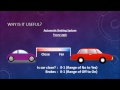• Fuzzy Logic Introduction

Fuzzy Logic Introduction This video was created for my Fuzzy Logic class to explain how I understand Fuzzy Logic and to give an example. From: Andrew Clemens Views: 46 1 ratings Time: 02:35 More in People & Blogs• mobile robot speed control with rotary encoder sensor using fuzzy logic

mobile robot speed control with rotary encoder sensor using fuzzy logic System specification: – microcontroller atmega 128 – motor DC pololu 29:1 350rpm – optical rotary encoder 36 counts per revolution – H-bridge mosfet driver motor – fuzzy logic control system… From: Rifqi Arridho Abid …How to add fuzzy logic rule to matlab simulink This video explains how to add the fuzzy rule to the simulink model in matlab. From: EDUCATIONS 360 Views: 108 0 ratings Time: 02:06 More in Education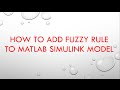• Self Balancing ROBOT with FUZZY LOGIC

Self Balancing ROBOT with FUZZY LOGIC Cooling system works great !!! I am looking for a good casing and waiting to see how long this lamp survive….. From: zubaer tanvir Views: 46 2 ratings Time: 00:29 More in Science & Technology• Mobile Robot Obstacle Avoidance Behavior Using Fuzzy Logic Controller

Mobile Robot Obstacle Avoidance Behavior Using Fuzzy Logic Controller From: AbdElkaReeM Muhtaseb Views: 14 0 ratings Time: 00:49 More in People & Blogs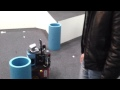• Mobile robot with Fuzzy logic – Lego Mindstorm

Mobile robot with Fuzzy logic – Lego Mindstorm Development of a mobile robot using fuzzy control, with the purpose to move to a desired position, dodging possible objects in the way. The robot was built using the LEGO Mindstorm platform… From: Eduardo Oest Views: 48 1 ratings Time: 00:25 …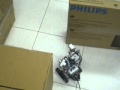• Fuzzy Logic Controller in Simulink MATLAB simulnk

Fuzzy Logic Controller in Simulink MATLAB simulnk Beginners Tutorial To demonstrate the fuzzy logic control capabilites of MATLAB we will simulate the example that was previously worked through. We will use the same input and output membershi… From: fendi setiabudi Views: 1093 1 ratings…• Getting Started with Fuzzy Logic Toolbox Part 1 MATLAB Video

Getting Started with Fuzzy Logic Toolbox Part 1 MATLAB Video From: mistv Views: 80 0 ratings Time: 05:06 More in Science & Technology• Intelligent Systems and Control Chap 2 Lecture 4 Introduction to Fuzzy Logic Control

Intelligent Systems and Control Chap 2 Lecture 4 Introduction to Fuzzy Logic Control From: Electrical Engineering Classroom Videos Views: 112 0 ratings Time: 01:01:05 More in People & Blogs• Fuzzy logic line follower Robot- UFO Creation

Fuzzy logic line follower Robot- UFO Creation Our 1st creation in sliit. From: infy zaz Views: 68 0 ratings Time: 00:51 More in People & Blogs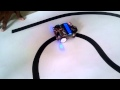• How To Use Fuzzy Logic with MS Access

How To Use Fuzzy Logic with MS Access Here is a pretty simple example of how to use fuzzy logic in MS Access. Pls feel free to Like , Comment and Subscribe. Other training videos are available on this channel. visit us today… From: Magik Systems Views: 56 0 ratings Time: 04:43 More in …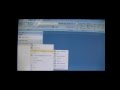• introduction of fuzzy logic

introduction of fuzzy logic From: Thesis Typing and Guidance Views: 10 1 ratings Time: 04:18 More in People & Blogs• obstacle avoidance bot – fuzzy logic

obstacle avoidance bot – fuzzy logic obstacle avoidance intellibrain mobot using fuzzy logic controller. From: James Kristopher Peña Views: 28 0 ratings Time: 04:55 More in People & Blogs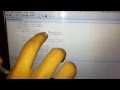• Fuzzy logic based navigation for mobile robot – M.Tech Robotics Project SRM University

Fuzzy logic based navigation for mobile robot – M.Tech Robotics Project SRM University A robot has been designed and fabricated which can autonomously navigate to predefined direction in unknown environment; scan the environment to generate SONAR map plus vision system for … From: SRM…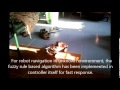• Servant Robot Navigation Using Gesture Recognition and Fuzzy Logic Sensor Fusion

Servant Robot Navigation Using Gesture Recognition and Fuzzy Logic Sensor Fusion Abstract—This paper proposes a new robot behavior where the servant robot runs alongside walls while it follows the human at a distance certain. The human shall give command using gestures… From: s101323561 …• Defining Fuzzy Logic with MATLAB

Defining Fuzzy Logic with MATLAB In this video is shown the fundamentals in defining mamdani style fuzzy logic using fuzzy toolbox in MATLAB software visit: www.delsim.org www.praktikum.delsim.org e-mail: delsim-lab@hotmail.com… From: Delsim Lab Views: 1110 0 ratings Time: 08:46 More in …• Fuzzy Logic 6 – How to win E3 2014

Fuzzy Logic 6 – How to win E3 2014 It’s Monday, and that means it’s time for another edition of Fuzzy Logic! Following on from last week’s Big Chat, Matt takes a look at what the big publishers and platform holders need to do… From: Bros With Fros Views: 15 2 ratings Time: …• fuzzy logic using matlab 3

fuzzy logic using matlab 3 ?? ????? relation using max–min and max–product composition. From: dodo4ever2100 Views: 187 0 ratings Time: 06:00 More in News & Politics• Mobile Robot Control Using Fuzzy Logic with MATLAB

Mobile Robot Control Using Fuzzy Logic with MATLAB From: Coretronix Labz Views: 616 2 ratings Time: 01:38 More in People & Blogs• iCat: Animal-inspired robot controller using hierarchical fuzzy logic controller

iCat: Animal-inspired robot controller using hierarchical fuzzy logic controller This video demonstrates cat-inspired robot controller which was implemented using Hierarchical Fuzzy Logic Controllers. Five major cat behaviours were replicated, these are (1) curiosity behaviour,… From: Emre…• tutorial on qtfuzzylite: a fuzzy logic control application in C++

tutorial on qtfuzzylite: a fuzzy logic control application in C++ This tutorial presents the main features of qtfuzzylite, a free open source fuzzy application to do fuzzy logic control in Linux, Mac and Windows. Available at http://www.fuzzylite.com. From: Juan Rada-Vilela Views: 12431 49…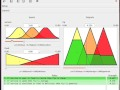• Getting Started with Fuzzy Logic Toolbox (Part 1)

Getting Started with Fuzzy Logic Toolbox (Part 1) Use Fuzzy Logic Toolbox™ to design fuzzy logic systems. From: MATLAB Views: 21651 40 ratings Time: 05:09 More in Science & Technology• How To Produce Perfect Half Boiled Egg By Using Fuzzy Logic

How To Produce Perfect Half Boiled Egg By Using Fuzzy Logic MATLAB is being used to do the fuzzy logic system. The input parameters are weight of egg, water temperature, and duration. The output parameter is rating of the half boiled egg. Rating 7… From: tan kai yin Views: 255 0 ratings…• Computer Science & Electronic Engineering “Fuzzy logic systems & robotics”: Prof Hani Hagras

Computer Science & Electronic Engineering “Fuzzy logic systems & robotics”: Prof Hani Hagras Professor Hani Hagras, from our School of Computer Science and Electronic Engineering, tells us more about intelligent robotics and fuzzy logic systems – these are intelligent systems…• Logic Pro Midi Template – Fuzzy Logic (How to Make Kyau & Albert Style)

Logic Pro Midi Template – Fuzzy Logic (How to Make Kyau & Albert Style) Download Now At: http://www.logictemplates.com/logic-pro-template-fuzzy-logic Logic Project Template Name: Fuzzy Logic Genre: Trance – Tech Duration: 1.56 BPM: 132 Creator: GMC DAW: Apple… From: Logic Pro Templates …• FUZZY LOGIC CONTROLLER FOR CONTROLLING DC MOTOR SPEED USING MATLAB APPLICATIONS

FUZZY LOGIC CONTROLLER FOR CONTROLLING DC MOTOR SPEED USING MATLAB APPLICATIONS Visit my blog for more details and code: http://umangjain25.blogspot.in/2012/12/dc-motor-speed-control-using-fuzzy.html. From: Umang Jain Views: 12215 11 ratings Time: 08:01 More in Science & Technology…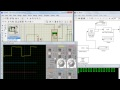• Mobile Robot IMU based on Sensor Fusion with Fuzzy Logic

Mobile Robot IMU based on Sensor Fusion with Fuzzy Logic This proyect fuses data from a 3-axis Accelerometer, 3 gyroscopes and a digital compass. Developed by: Carlos A. Monsalve Julian C. Villa Juan C. Gómez Hardware used: MMA7360 Accelerometer… From: Juan Camilo Gomez Cadavid Views: 544 …• Dynamic Gait Adaptation of ModRED modular robot using fuzzy logic control

Dynamic Gait Adaptation of ModRED modular robot using fuzzy logic control Dynamic gait adaptation of the ModRED modular robot using a fuzzy logic controller. The objective the robot is to align itself with goal direction that is specified externally (either by a… From: cmanticlab Views: 260 …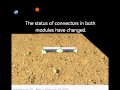• Small Mobile Robot for Wall-Following using Embeded Fuzzy Logic Controller

Small Mobile Robot for Wall-Following using Embeded Fuzzy Logic Controller From: Fahmizal ST Views: 440 3 ratings Time: 02:22 More in Science & Technology• Simulink tutorial – using Fuzzy Logic

Simulink tutorial – using Fuzzy Logic This is Simulink tutorial: Fuzzy Logic. This video teaches you how to use a Fuzzy Object in Simulink. The code can be found in the tutorial section in http://www.eeprogrammer.com/. More engineering… From: eeprogrammer Views: 16696 37 ratings Time: …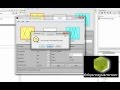• http://www.fuzzy-logic.com – How to install ExisEcho on your computer

http://www.fuzzy-logic.com – How to install ExisEcho on your computer This short 4 minute video shows you how to download and install ExisEcho from our website at http://www.fuzzy-logic.com. From: exisllc Views: 78 0 ratings Time: 04:01 More in Howto & Style• soft computing lecture – hour 18: Fuzzy Logic

soft computing lecture – hour 18: Fuzzy Logic video lecture series covering theoretical and application areas of soft computing was recorded at ABV-IIITM Gwalior. complete index, video and download link available at http://rkala.in/softcomputi… From: rahul kala Views: 1820 2 ratings Time: …• Fuzzy Logic Examples using Matlab part 3 (Admire Solution).avi

Fuzzy Logic Examples using Matlab part 3 (Admire Solution).avi Admire Solution. From: Karthic Easwaran Views: 9660 10 ratings Time: 03:48 More in Education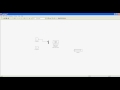• What is Fuzzy logic: An introduction

What is Fuzzy logic: An introduction Website:http://urbanschool.in/index.htm Facebook: https://www.facebook.com/urbanschool.in Email: satendra.svnit@gmail.com For More log on to http://urbanschool.in/tutorials.html. From: satendra kumar Views: 15952 0 ratings Time: 06:11 More in Education…• How to park a car with Fuzzy Logic

How to park a car with Fuzzy Logic parking a car in a given parkin-lot with Matlab Simulink and a fuzzy logic controller. From: Nick Fischer Views: 3788 6 ratings Time: 00:58 More in Autos & Vehicles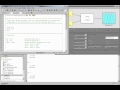• two wheels balancing line tracer robot using fuzzy logic control.avi

two wheels balancing line tracer robot using fuzzy logic control.avi Best Student paper awards IES 2011. From: abiinindra Views: 656 2 ratings Time: 00:46 More in People & Blogs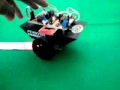• Fuzzy Logic Control of Single Link Flexible Joint Robot Manipulator

Fuzzy Logic Control of Single Link Flexible Joint Robot Manipulator Fuzzy Logic Control of Single Link Flexible Joint Robot Manipulator. From: smlkyz Views: 1008 2 ratings Time: 00:52 More in Education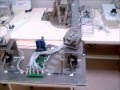• Fuzzy Logic: An Introduction

Fuzzy Logic: An Introduction This video introduces fuzzy logic, including the basics of fuzzy sets, fuzzy rules and how these are combined in decision making. From: cisieee Views: 54282 340 ratings Time: 04:48 More in Education• Robot ProBot 128 controlled by fuzzy logic• PID using Fuzzy Logic Toolbox.wmv

PID using Fuzzy Logic Toolbox.wmv This video shows how to use the Fuzzy logic Toolbox from Simulink to implement a PID regulator. rjuarez7@gmail.com. From: Rafael Juarez Views: 12438 16 ratings Time: 00:52 More in Science & Technology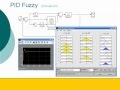• Quantum~Fuzzy Logic based control of a mobile robot

Quantum~Fuzzy Logic based control of a mobile robot A Fuzzy controlled mobile robot. The Fuzzy Control is based on a developed transformation of Quantum logic into a Fuzzy model thus allowing application of a Fuzzy control using the Quantum model. From: kainil Views: 1251 0 ratings Time: …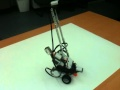• TORCS Fuzzy Logic Robot

TORCS Fuzzy Logic Robot A driving robot programmed in C++ by me and my friend as a final assignment for fuzzy logic course at Faculty of Mathematics and Computer Science, Adam Mickiewicz University in Pozna?, Poland…. From: NadrinVilo Views: 6371 11 ratings Time: 01:57 More in Science…• Fuzzy Logic and Robotic Control with Compact Rio

Fuzzy Logic and Robotic Control with Compact Rio A robot that parks itself using NI Compact RIO and the Fuzzy Logic Toolkit. Programmed in LabVIEW, the Fuzzy Logic determines the direction of movement and the angle of turn of the robot. This… From: nifirstrobotics Views: 359 1 ratings Time:…• Module 2 Lecture 4 Introduction to Fuzzy Logic Control

Module 2 Lecture 4 Introduction to Fuzzy Logic Control Lectures by Prof. Laxmidhar Behera, Department of Electrical Engineering, Indian Institute of Technology, Kanpur. For more details on NPTEL visit http://nptel.iitm.ac.in. From: nptelhrd Views: 42088 34 ratings Time: 01:01:05 More in …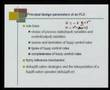• Fuzzy Logic and Robotic Control with NI Compact-RIO

Fuzzy Logic and Robotic Control with NI Compact-RIO In this video, we have created a robot which parks itself using NI Compact RIO and the Fuzzy Logic Toolkit. Programmed in LabVIEW, the Fuzzy Logic determines the direction of movement and the… From: niasean Views: 19401 12 ratings Time: …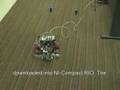(Visited 782 times, 1 visits today)